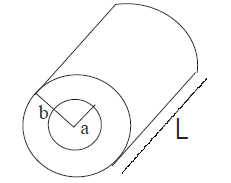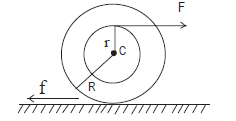# Rotational Dynamics Numericals

In this page we have Rotational Motion Problems for JEE and NEET . Hope you like them and do not forget to like , social share and comment at the end of the page.
Question 1
A uniform hollow cylinder has a density $\rho$, a length L, an inner radius a, and outer radius b. Show that its moment of inertia about the axis of cylinder is $I=\frac {1}{2} \pi \rho L (b^4 -a^4)=\frac {1}{2} M(b^2 + a^2)$, where M is the mass of the cylinder.Question 2
Starting from rest, a sphere rolls down a $30^0$ incline. What is the minimum value of coefficient of Static friction if there is to be no slipping?

Question 3
A spool of mass M is resting on a horizontal surface. The spool has moment of inertia $MG^2c$ about its axis of symmetry. The spool is subjected to the rightward horizontal force of magnitude F, applied at a distance r above the axis.(a) Show if there is no slippage between spools and surrounding surface, a leftward frictional force $f=\frac {F(G^2c -rR)}{G^2c +R^2}$ must act on the spool (b) Show that the required fractional force f has the value zero for the particular $r_0$ of the distance r.

Question 4
A circular disc of mass M and radius R revolves about its own axis with angular velocity $\omega$
Find out following
(a) Moment of Inertia about its own axis
(b) Kinetic energy of rotation
(c) Angular momentum of the disc about its own axis
(d) If a another disc of mass M and radius R is placed on this disk such that axis of rotation is along the same line, what will be the final Angular velocity of the system

Question 5
A rod of length L and Mass M lies on a frictionless horizontal table. It is free to move in any way on the table. A small body of mass m moving with a velocity u collides in elastically with the rod .
(a) Find the velocity of the centre of mass of the rod
(b) Find the angular velocity of the rod about centre of mass

Question 6
A uniform disc of Radius R and mass M is spinned to the angular velocity ω0 and then it is placed on a horizontal surface .The coefficient of friction between the disc and surface is μ. The pressure exerted by the disc on the surface can be regarded as uniform
(a) Find the moment of inertia of the disc about the rotating axis
(b) Find the angular deceleration of the disc
(c) Find the time in which disc will come to rest
(d) The kinetic energy of the disc t sec after the disc is placed on the surface

Question 7
A uniform thin bar of mass M kg and length L is bent to make a square. Calculate its moment of inertia about an axis passing through the centre of mass and perpendicular to the plane of square